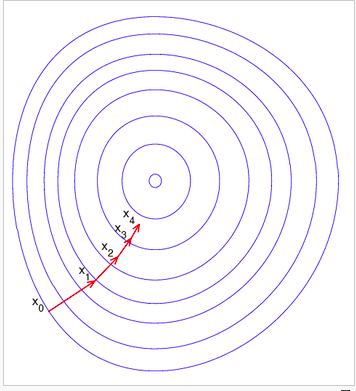# logistic回归详解（三）：梯度下降训练方法

http://blog.csdn.net/bitcarmanlee/article/details/51165444中，我们已经对logistic回归的cost function做了完整的推导。如果是单个样本，其损失函数为：

cost(hθ(x),y)=yilog(hθ(x))(1yi)log(1hθ(x))

## 1.梯度下降的原理

b=aγF(a)$\mathbf{b}=\mathbf{a}-\gamma\nabla F(\mathbf{a})$

xn+1=xnγnF(xn), n0$\mathbf{x}_{n+1}=\mathbf{x}_n-\gamma_n \nabla F(\mathbf{x}_n),\ n \ge 0$

F(x0)F(x1)F(x2)$F(\mathbf{x}_0)\ge F(\mathbf{x}_1)\ge F(\mathbf{x}_2)\ge \cdots$,## 2.对损失函数求导并得出迭代公式

J(θ)=cost(hθ(x),y)=yilog(hθ(x))(1yi)log(1hθ(x))
，则：
θJ(θj)=(y1g(θTx)(1y)11g(θTx))θjg(θTx)=(y1g(θTx)(1y)11g(θTx))g(θTx)(1g(θTx))θjθTx)=(y(1g(θTx))(1y)g(θTx))xj=(hθ(x)y)xj

θj:=θjα(hθ(xi)yi)xij

θj:=θjimα(hθ(xi)yi)xij

## 3.迭代公式向量化（vectorization)

θj:=θjimα(hθ(xi)yi)xij

X=x(1)x(2)x(m)=x(1)0x(2)0x(m)0x(1)1x(2)1x(m)1x(1)nx(2)nx(m)n

y=y(1)y(2)y(m)

θ=θ0θ1θn

xθ$x\cdot\theta$的乘积记为A，有：

A=xθ=x(1)0x(2)0x(m)0x(1)1x(2)1x(m)1x(1)nx(2)nx(m)nθ0θ1θm=θ0x(1)0θ0x(2)0θ0x(m)0θ1x(1)1θ1x(2)1θ1x(m)1θnx(1)nθnx(2)nθnx(m)n

hθ(x)y$h_{\theta}(x)-y$记为E:

E=hθ(x)y=g(A1)y1g(A2)y2g(Am)ym=e1e2em=g(A)y

θ0:=θ0imα(hθ(xi)yi)xi0=θ0αime(i)x(i)0=θ0α(x(1)0,x(2)0,,x(n)0)E

θj:=θjα(x(1)j,x(2)j,,x(n)j)E

θ0θ1θm:=θ0θ1θmαx(1)0x(1)1x(1)nx(2)0x(2)1x(2)nx(m)0x(m)1x(m)nE=θαxTE

θ=θαxTE

## 4.几点需要注意的事项

1.x的矩阵表示方式里，上边的范围是m,表示样本的数量，下边的范围是n，表示每个样本变量的维度。整个样本矩阵的大小是m*n。
2.如何快速理解θ$\theta$的迭代公式？我自己总结的一个小技巧：
θ$\theta$表示每一维特征的权重，所以它是n*1的矩阵。xT$x^T$是n*m，E是m*1,这两个矩阵相乘，刚好得到一个n*1的矩阵，跟θ$\theta$的大小是相吻合的！

## 5.批量梯度下降（Batch Gradient Descent）与随机梯度下降(Stochastic Gradient Descent SGD)

θ=θαxTE

BGD是一次训练带入所有样本，SGD则是每来一次样本进行一次计算：
θj:=θj+α(yihθ(xi))xij

i表示是第i个样本，j表示样本第j个维度。
SGD是通过每个样本来迭代更新。如果样本的数量很多，有可能才迭代了一小部分样本，就已经得到了θ$\theta$的解。所以SGD的收敛速度可能比BGD要快，而且运算量小。但是SGD的问题是每次迭代并不是全局最优解的方向，尤其是遇到噪声数据，影响会比较大。有的时候SGD在最优解附近会存在比较明显的锯齿震荡现象，即损失函数的值会在最优解附近上下震荡一段时间才最终收敛。